2018俄罗斯世界杯32强各支球队均公布了初选大名单，西班牙、葡萄牙、摩洛哥、法国、巴西、阿根廷、英格兰、冰岛、哥斯达黎加、瑞典和塞内加尔共11支球队则提前公布了最终的23人大名单，32强剩余的球队将在6月4日前提交最终的23人大名单。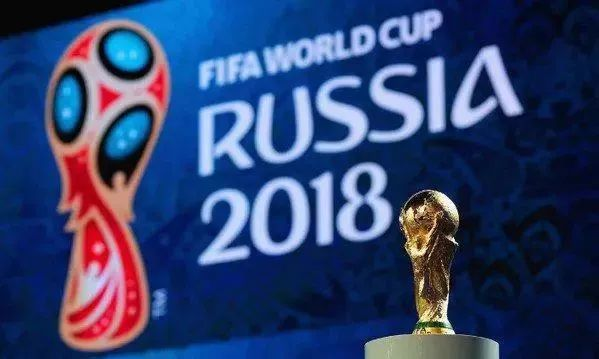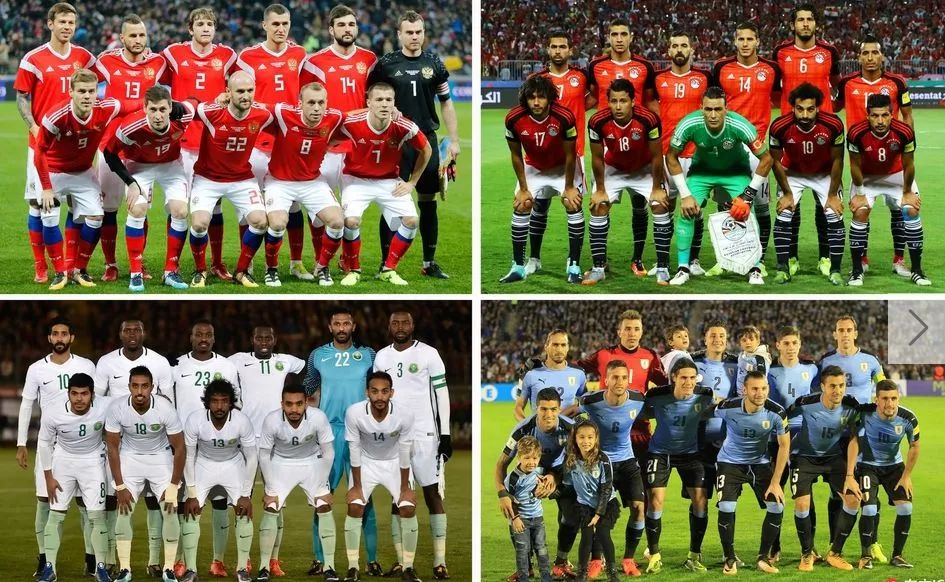门将：埃尔-哈达里(布赖代合作)、-希纳维(开罗国民)、埃克拉米(开罗国民)、阿瓦德(伊斯梅利);

后卫：阿赫梅德-法蒂(开罗国民)、埃尔穆哈马迪(阿斯顿维拉)、奥马尔-贾比尔(洛杉矶)、-沙菲(哈萨征服)、萨米尔(开罗国民)、赫加齐(西布朗)、贾布尔(西布朗)、阿什拉夫(开罗国民)、哈菲兹(朗斯)、塔雷克(奥兰多城)、汉姆迪(扎马莱克);

中场：希卡巴拉(布赖代先锋)、阿布杜拉-赛义德(古比斯)、埃尔内尼(阿森纳)、塔雷克-哈默德(扎马莱克)、卡拉巴(吉达联合)、-哈桑(卡斯帕萨)、索比(斯托克城)、穆尔西(维冈竞技)、瓦尔达(阿特罗米托斯)、阿齐兹(扎马莱克)；

前锋：萨拉赫(利物浦)、穆赫辛(开罗国民)、马哈古卜(布拉加)、戈马(马斯里)。

门将：阿金费耶夫(莫斯科中央陆军)、加布洛夫(布鲁日)、扎纳耶夫(喀山红宝石)、鲁涅夫(圣彼得堡泽尼特)；

后卫：格拉纳特(喀山红宝石)、伊格纳舍维奇(莫斯科中央陆军）、库德里亚绍夫(喀山红宝石)、库捷波夫(莫斯科斯巴达)、诺伊施塔特(费内巴切)、劳施(莫斯科迪纳摩)、塞梅诺夫(格罗兹尼)、斯莫尔尼科夫(圣彼得堡泽尼特)、马里奥-费尔南德斯(莫斯科中央陆军)；

中场：加辛斯基(克拉斯诺达尔)、戈洛温(莫斯科中央陆军)、扎戈耶夫(莫斯科中央陆军)、叶罗欣(圣彼得堡泽尼特)、日尔科夫(圣彼得堡泽尼特)、佐布宁(莫斯科斯巴达)、库兹亚耶夫(圣彼得堡泽尼特)、安东-米兰丘克(莫斯科火车头)、萨梅多夫(莫斯科斯巴达)、塔沙耶夫(莫斯科迪纳摩)、切里舍夫(比利亚雷亚尔)；

前锋：久巴(图拉兵工厂)、阿列克谢-米兰丘克(莫斯科火车头)、斯莫洛夫(克拉斯诺达尔)、恰诺夫(莫斯科中央陆军)。

门将：卡尔尼(吉达联合)、奥威斯(吉达国民)、莫塞莱姆(吉达国民)、马尤夫(利雅得新月)；

后卫：哈尔比(吉达国民)、沙赫拉尼(利雅得新月)、布雷克(利雅得新月)、萨义德-穆瓦拉德(吉达国民)、豪萨维(吉达国民)、穆塔兹-豪萨维(吉达国民)、奥马尔-豪萨维(利雅得胜利)、贾赫法利(利雅得新月)、布莱希(法特赫)；

中场：海巴里(利雅得青年)、海布里(利雅得新月)、奥塔伊夫(利雅得新月)、贾西姆(吉达国民)、莫加维(吉达国民)、法拉吉(利雅得新月)、阿比德(利雅得新月)、卡诺(利雅得新月)、巴赫布里(利雅得青年)、塞勒姆(达曼协作)、达瓦萨里(比利亚雷亚尔)、谢赫里(莱加内斯)；

门将：穆斯莱拉(加拉塔萨雷)、马丁-席尔瓦(瓦斯科达迦马)、坎帕尼亚(独立)；

后卫：戈丁(马德里竞技)、科茨(葡萄牙体育)、希门尼斯(马德里竞技)、佩雷拉(波尔图)、加斯顿-席尔瓦(独立)、卡塞雷斯(拉齐奥)、巴雷拉(佩纳罗尔)；

中场：南德斯(博卡青年)、托雷拉(桑普多利亚)、贝西诺(国际米兰)、巴尔韦尔德(拉科鲁尼亚)、本坦库尔(尤文图斯)、卡洛斯-桑切斯(蒙特雷)、德阿拉斯卡埃塔(克鲁塞罗)、拉克索尔特(热那亚)、克里斯蒂安-罗德里格斯(佩纳罗尔)、乌雷塔维斯卡亚(蒙特雷)、洛代罗(西雅图海湾人)、加斯顿-拉米雷斯(桑普多利亚)；

前锋：斯图亚尼(赫罗纳)、戈麦斯(塞尔塔)、卡瓦尼(巴黎圣日耳曼)、苏亚雷斯(巴塞罗那)。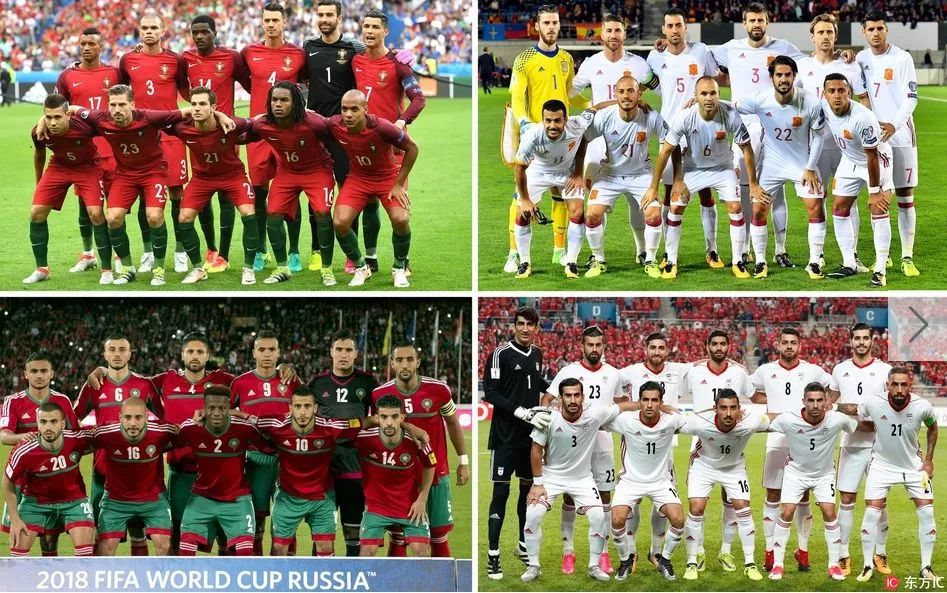门将：贝兰万德（波斯波利斯）、马扎赫里（佐布阿汉）、阿比德扎德（葡萄牙马里蒂莫）

后卫：雷扎埃安（比利时奥斯坦德）、普拉利甘吉（卡塔尔萨德）、蒙塔泽里（德黑兰独立）、雷扎哈扎德（帕迪代）、米拉德穆罕默迪（俄罗斯格罗兹尼艾哈迈德）、马吉德侯赛尼（德黑兰独立）、切什米（德黑兰独立）

中场：埃扎托拉希（俄罗斯彼尔姆安卡）、哈吉萨菲（希腊奥林匹亚科斯）、易卜拉西米（德黑兰独立）、舒贾埃（雅典AEK）、德贾加（英格兰诺丁汉森林）

前锋：贾汉巴赫什（荷兰阿尔克马尔）、阿兹蒙（俄罗斯喀山红宝石）、塔雷米（卡塔尔加拉法）、戈多斯（瑞典厄斯特松德）、托拉比（赛帕）、戈利扎德（赛帕）、古钱内贾德（荷兰海伦芬）、安萨里法德（希腊奥林匹亚科斯）、阿米里（波斯波利斯）

门将：穆尼尔-穆罕默迪(努曼西亚)、布努(赫罗纳)、塔格诺提(丹吉尔)；

后卫：贝纳蒂亚(尤文图斯)、塞萨(狼队)、达科斯塔(伊斯坦布尔)、阿什拉夫(皇家马德里)、迪拉尔(费内巴切)、巴诺恩(卡萨布兰卡拉贾)、门迪耶尔(里尔)；

中场：本纳赛尔(卡昂)、卡塞拉(标准列日)、艾哈迈迪(费耶诺德)、贝尔汉达(加拉塔萨雷)、齐耶赫(阿贾克斯)、费萨尔(赫塔费)、小阿姆拉巴特(费耶诺德)、布苏法(阿布扎比半岛)、阿里(沙尔克04)；

前锋：布哈杜兹(圣保利)、阿姆拉巴特(莱加内斯)、埃尔卡比(贝尔卡内)、布塔伊卜(马拉蒂亚体育)。

门将：安东尼-洛佩斯(里昂)，贝托(哥兹塔比)，帕特里西奥(葡萄牙体育)

后卫：布鲁诺-阿尔维斯(流浪者)，塞德里克-苏亚雷斯(南安普顿)，丰特(大连一方)，马里奥-鲁伊(那不勒斯)，佩佩(贝西克塔斯)，格雷罗(多特蒙德)，里卡多-佩雷拉(波尔图)，鲁本-迪亚斯(本菲卡)

中场：阿德里安-席尔瓦(莱斯特城)，布鲁诺-费尔南德斯(葡萄牙体育)，若昂-马里奥(西汉姆联)，穆蒂尼奥(摩纳哥)，曼努埃尔-费尔南德斯(莫斯科火车头)，威廉-卡瓦略(葡萄牙体育)

前锋：安德烈-席尔瓦(AC米兰)，伯纳多-席尔瓦(曼城)，C罗(皇家马德里)，热尔松-马丁斯(葡萄牙体育)，格德斯(瓦伦西亚)，夸雷斯马(贝西克塔斯)。

后卫：皮克(巴萨)、拉莫斯(皇马)、阿斯皮利奎塔(切尔西)、纳乔(皇马)、奥德里奥索拉(皇家社会)、阿尔巴(巴萨)、卡瓦哈尔(皇马)、蒙雷亚尔(阿森纳)；

中场：席尔瓦(曼城)、布斯克茨(巴萨)、阿森西奥(皇马)、伊涅斯塔(巴萨)、伊斯科(皇马)、萨乌尔(马竞)、蒂亚戈(拜仁)、科克(马竞)；

前锋：卢卡斯-巴斯克斯(皇马)、阿斯帕斯(塞尔塔)、罗德里戈(瓦伦西亚)、迭戈-科斯塔(马竞)。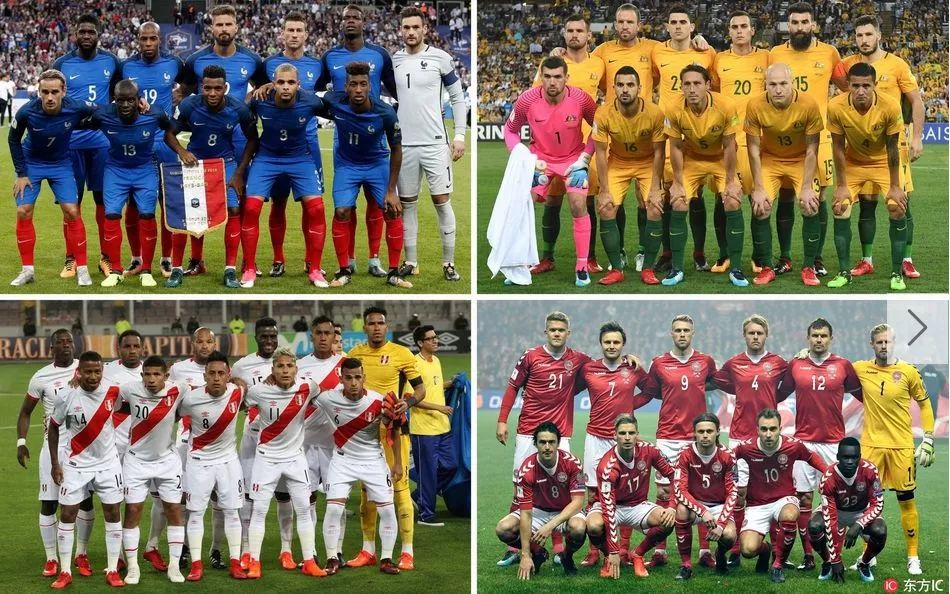后卫：门迪(曼城)、西迪贝(摩纳哥)、卢卡斯-埃尔南德斯(马竞)、金彭贝(巴黎圣日耳曼)、乌姆蒂蒂(巴萨)、瓦拉内(皇马)、拉米(马赛)、帕瓦尔(斯图加特)

中场：坎特(切尔西)、马图伊迪(尤文)、博格巴(曼联)、恩宗齐(塞维利亚)、托利索(拜仁)

前锋：费基尔(里昂)、奥斯曼-登贝莱(巴萨)、吉鲁(切尔西)、格列兹曼(马竞)、姆巴佩(巴黎圣日耳曼)、托万(马赛)、勒马尔(摩纳哥)。

门将：马修-瑞安(布莱顿)，布拉德-琼斯(费耶诺德)，武科维奇(亨克)；

后卫：塞恩斯伯里(苏黎世草蜢)，尤尔曼(水原三星)，德格内克(横滨水手)，里斯登(西悉尼流浪者)，比伊奇(布尔萨体育)，梅雷迪思(米尔沃尔)，卡拉契奇(萨格勒布火车头)；

中场：耶迪纳克(阿斯顿维拉)，穆伊(哈德斯菲尔德)，罗吉奇(凯尔特人)，隆戈(女王公园巡游者)，杰克逊-欧文(赫尔城)，布里兰特(悉尼FC)，佩特拉托斯(纽卡斯尔喷气机)，米利甘(吉达国民)

前锋：蒂姆-卡希尔(米尔沃尔)，尤里奇(卢塞恩)，马修-莱基(柏林赫塔)，特洛伊西(墨尔本胜利)，阿尔扎尼(墨尔本城)，纳鲍特(浦和红钻)，卢卡维奇亚(海法马卡比)，克鲁泽(波鸿)。

门将：小舒梅切尔(莱斯特城)、怀诺乌(布隆德比)、罗斯尔(哈德斯菲尔德)、汉森(中日德兰)；

后卫：克亚尔(塞维利亚)、别兰(布伦特福德)、赞卡-约根森(哈德斯菲尔德)、克里斯滕森(切尔西)、拉森(乌迪内斯)、杜尔米西(皇家贝蒂斯)、维斯特高(门兴格拉德巴赫)、博伊莱森(哥本哈根)、达尔斯高(布伦特福德)、克努森(伊普斯维奇)、安克尔森(哥本哈根)；

中场：埃里克森(热刺)、德莱尼(云达不莱梅)、奎斯特(哥本哈根)、舍内(阿贾克斯)、莱拉格(波尔多)、延森(罗森博格)、赫伊别尔(南安普顿)、克隆-德利(拉科鲁尼亚)、沃斯(塞尔塔)、马蒂亚斯-延森(北西兰)；

前锋：约根森(费耶诺德)、科内柳斯(亚特兰大)、本特纳(罗森博格)、西斯托(塞尔塔)、菲舍尔(哥本哈根)、波尔森(RB莱比锡)、布莱斯维特(波尔多)、斯科夫(哥本哈根)、多尔贝尔(阿贾克斯)、佐霍雷(卡迪夫城)。

门将：卡塞达(慕斯斯帕尔体育)、何塞-卡瓦略(UTC)、加莱塞(贝拉克鲁斯)；

后卫：艾布拉姆(萨斯菲尔德)、阿德文库拉(罗伯斯)、阿劳霍(利马联盟)、科尔佐(秘鲁体育大学)、洛约拉(梅尔加)、克里斯蒂安-拉莫斯(贝拉克鲁斯)、阿尔伯托-罗德里格斯(水晶体育)、圣玛里亚(普埃布拉)、特劳科(弗拉门戈)；

中场：佩德罗-阿基诺(罗伯斯)、卡塔赫纳(贝拉克鲁斯)、奎瓦(圣保罗)、弗洛雷斯(奥尔堡)、佩尼亚(格拉纳达)、塔皮亚(费耶诺德)、尤顿(奥兰多城)；

前锋：卡里略(沃特福德)、法尔范(莫斯科火车头)、乌尔塔多(维多利亚)、波洛(波特兰伐木者)、鲁伊迪亚斯(莫雷利亚)。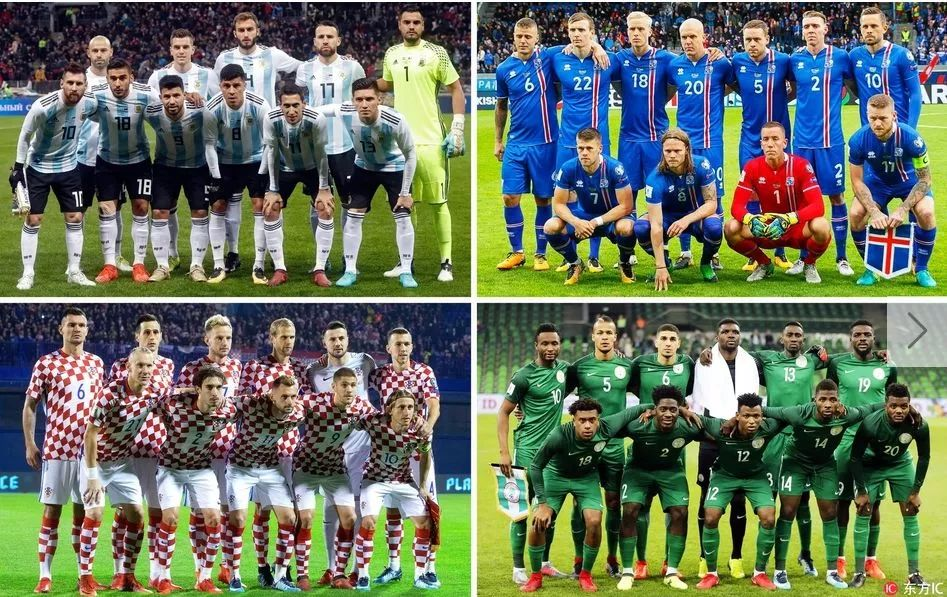后卫：梅尔卡多(塞维利亚)、奥塔门迪(曼城)、法西奥(罗马)、罗霍(曼联)、阿库尼亚(葡萄牙体育)、塔利亚菲科(阿贾克斯)、安萨尔迪(都灵)；

中场：马斯切拉诺(河北华夏幸福)、兰奇尼(西汉姆联)、梅萨(独立)、比格利亚(AC米兰)、巴内加(塞维利亚)、洛赛尔索(巴黎圣日耳曼)、迪马利亚(巴黎圣日耳曼)、帕文(博卡青年)、萨尔维奥(本菲卡)；

前锋：迪巴拉(尤文图斯)、梅西(巴塞罗那)、阿圭罗(曼城)、伊瓜因(尤文图斯)。

门将：苏巴西奇(摩纳哥)、L-卡利尼奇(根特)、利瓦科维奇(萨格勒布迪纳摩)；

后卫：乔尔卢卡(莫斯科火车头)、维达(贝西克塔斯)、斯特里尼奇(桑普多利亚)、洛夫伦(利物浦)、弗尔萨利科(马德里竞技)、皮瓦里奇(基辅迪纳摩)、耶德瓦伊(勒沃库森)、米特罗维奇(布鲁日)、卡莱塔-卡尔(萨尔茨堡红牛)；

中场：莫德里奇(皇家马德里)、拉基蒂奇(巴塞罗那)、科瓦契奇(皇家马德里)、巴德利(佛罗伦萨)、布罗佐维奇(国际米兰)、布拉达里奇(里耶卡)；

前锋：曼朱基奇(尤文图斯)、佩里西奇(国际米兰)、卡利尼奇(AC米兰)、克拉马里奇(霍芬海姆)、皮亚察(沙尔克04)、雷比奇(法兰克福)。

门将：埃曾瓦(安耶巴)、阿克佩伊(茨普帕联)、乌佐霍(拉科鲁尼亚)、阿吉博耶(普拉托联)；

后卫：埃孔(布尔萨体育)、巴洛贡(美因茨)、埃纳(赫尔城)、奥梅罗(卡斯帕萨)、伊多武(阿姆卡尔)、阿瓦泽姆(南特)、谢胡(布尔萨体育)、埃切吉莱(色格拉布鲁日)、埃布埃希(海牙)、埃泽(普罗迪夫火车头)；

中场：米克尔(天津泰达)、奥纳齐(特拉布宗体育)、奥古(贝尔谢巴工人)、恩迪迪(莱斯特城)、阿格博(标准列日)、埃特博(拉斯帕尔马斯)、奥比(都灵)、阿古(布尔萨体育)；

前锋：伊哈洛(长春亚泰)、穆萨(莫斯科中央陆军)、摩西(切尔西)、伊沃比(阿森纳)、伊希纳乔(莱斯特城)、西蒙(根特)、洛科萨(卡诺支柱)、恩万科沃(克罗托内)。

前锋：西古拉松(罗斯托夫)、古德蒙德松(PSV埃因霍温)、芬博阿松(奥格斯堡)、博瓦松(雷丁)

中场：古德蒙德松(伯恩利)、特劳斯塔松(马尔默)、贡纳尔松(卡迪夫城)、O-斯库拉松(卡拉比克体育)、西于尔兹松(埃弗顿)、哈尔弗雷德松(乌迪内斯)、比亚尔纳松(阿斯顿维拉)、吉斯拉松(桑德豪森)

后卫：斯库拉松(洛克伦)、马格努松(布里斯托城)、埃约尔夫松(索菲亚列夫斯基)、阿纳松(阿伯丁)、因加松(罗斯托夫)、拉格纳-西于尔兹松(罗斯托夫)、弗里奥荣松(瓦勒伦加)、塞瓦尔松(瓦鲁尔)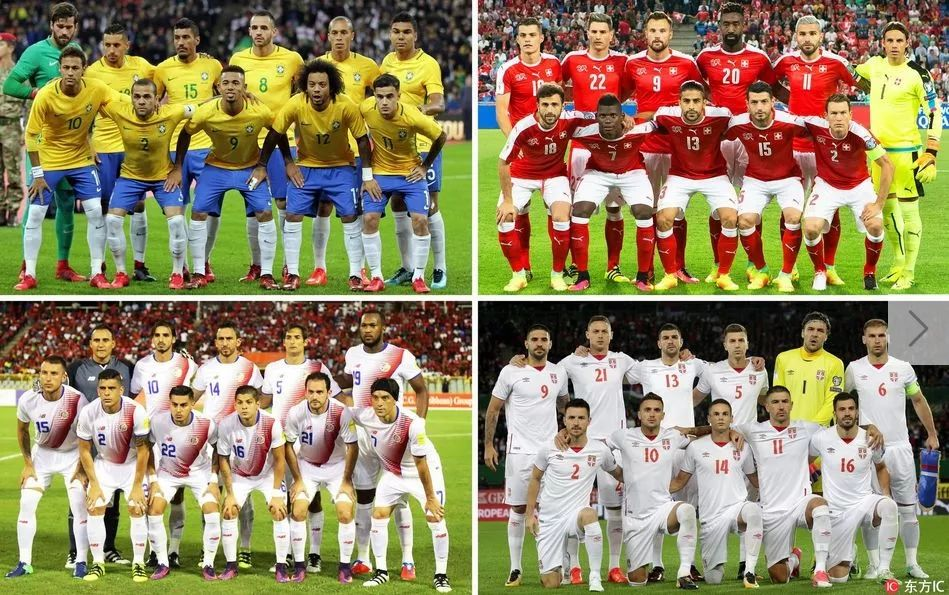后卫：达尼洛(曼城)、法格纳(科林蒂安)、马塞洛(皇家马德里)、菲利佩(马德里竞技)；蒂亚戈-席尔瓦(巴黎圣日耳曼)、马尔基尼奥斯(巴黎圣日耳曼)、米兰达(国际米兰)、杰罗梅尔(格雷米奥)；

中场：卡塞米罗(皇家马德里)、费尔南迪尼奥(曼城)、保利尼奥(巴塞罗那)、弗雷德(顿涅茨克矿工)、奥古斯托(北京中赫国安)、库蒂尼奥(巴塞罗那)、威廉(切尔西)、道格拉斯-科斯塔(尤文图斯)；

前锋：内马尔(巴黎圣日耳曼)、泰森(顿涅茨克矿工)、热苏斯(曼城)、菲尔米诺(利物浦)。

门将：布尔基(多特)、格雷戈-科贝尔(霍芬海姆)、姆沃戈(RB莱比锡)、索默(门兴)

后卫：阿坎季(多特)、朱鲁(安塔利亚体育)、埃尔维迪(门兴)、迈克尔-朗(巴塞尔)、利希施泰纳(尤文)、穆班杰(图卢兹)、里卡多-罗德里格斯(米兰)、法比安-舍尔(拉科)、西尔万-威德默(乌迪内斯)

中前场：贝赫拉米(乌迪内斯)、德尔米奇(门兴)、哲马伊利(博洛尼亚)、恩博洛(沙尔克04)、费尔南德斯(西汉姆联)、热尔松-费尔南德斯(法兰克福)、弗罗伊勒(亚特兰大)、加夫拉诺维奇(萨格勒布迪纳摩)、塞费罗维奇(本菲卡)、沙奇里(斯托克城)、扎卡(阿森纳)、扎卡里亚(门兴)、史蒂芬-祖伯(霍芬海姆)

门将：纳瓦斯(皇家马德里)、彭伯顿(阿拉胡埃伦斯)、莫雷拉(埃雷迪亚诺)；

后卫：甘博亚(凯尔特人)、伊恩-史密斯(北雪平)、马塔里塔(纽约城)、奥维多(桑德兰)、杜阿尔特(西班牙人)、詹卡洛-冈萨雷斯(博洛尼亚)、卡尔沃(明尼苏达联)、沃森(温哥华白浪)、阿科斯塔(多拉多斯)；

中场：古兹曼(波特兰伐木者)、特赫达(洛桑体育)、博尔赫斯(拉科鲁尼亚)、阿佐菲法(埃雷迪亚诺)、罗德尼-华莱士(纽约城)、鲁伊斯(葡萄牙体育)、科林德雷斯(萨普里萨)、博拉尼奥斯(萨普里萨)；

前锋：贝内加斯(萨普里萨)、乔尔-坎贝尔(皇家贝蒂斯)、乌雷尼亚(洛杉矶FC)。

门将：斯托伊科维奇(贝尔格莱德游击)、德米特罗维奇(埃瓦尔)、拉伊科维奇(特拉维夫马卡比)、约万诺维奇(奥胡斯费马)

后卫：卢卡维纳(比利亚雷亚尔)、科拉罗夫(罗马)、罗迪克(贝尔格莱德红星)、伊万诺维奇(泽尼特)、斯帕吉奇(克拉斯诺达尔)、纳斯塔西奇(沙尔克04)、托西奇(广州富力)、维利科维奇(不莱梅)、米伦科维奇(佛罗伦萨)

中场：米利沃杰维奇(水晶宫)、马蒂奇(曼联)、格鲁伊奇(利物浦)、利亚伊奇(都灵)、米林科维奇(拉齐奥)、塔迪奇(南安普顿)、科斯蒂奇(汉堡)、加齐诺维奇(法兰克福)、日夫科维奇(本菲卡)、拉多尼奇(贝尔格莱德红星)、马克西莫维奇(瓦伦西亚)

前锋：米特罗维奇(富勒姆/纽卡斯尔联)、普利约维奇(塞萨洛尼基)、约维奇(法兰克福)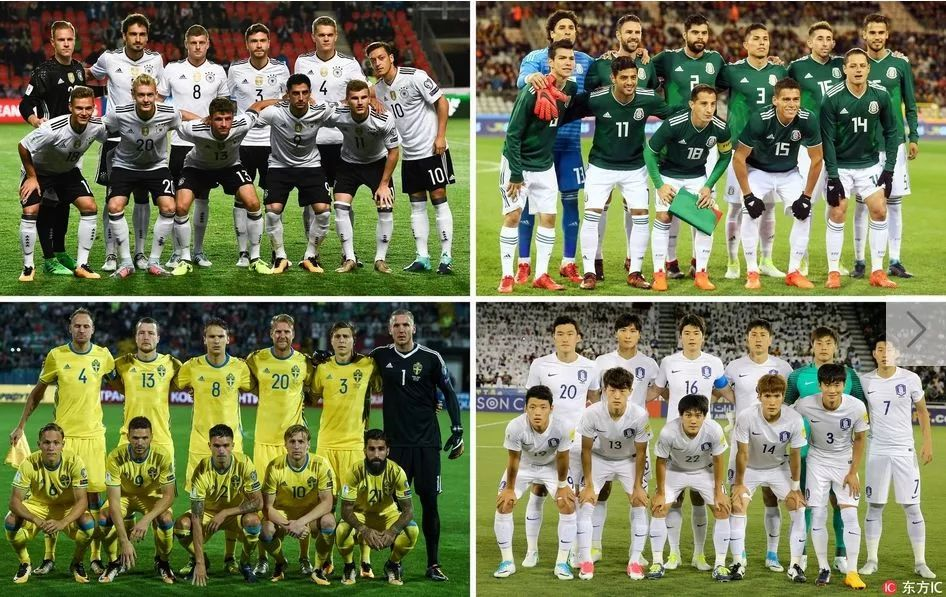门将：诺伊尔(拜仁)、特尔施特根(巴萨)、莱诺(勒沃库森)、特拉普(巴黎)

后卫：基米希(拜仁)、博阿滕(拜仁)、胡梅尔斯(拜仁)、聚勒(拜仁)、赫克托(科隆)、约纳坦-塔(勒沃库森)、金特尔(门兴)、普拉滕哈特(柏林赫塔)、吕迪格(切尔西)

中前场：布兰特(勒沃库森)、德拉克斯勒(巴黎)、戈麦斯(斯图加特)、格雷茨卡(沙尔克)、京多安(曼城)、赫迪拉(尤文图斯)、克罗斯(皇马)、托马斯-穆勒(拜仁)、厄齐尔(阿森纳)、彼得森(弗赖堡)、罗伊斯(多特)、鲁迪(拜仁)、萨内(曼城)、维尔纳(莱比锡)

后卫：埃德森-阿尔瓦雷斯(美洲)、阿拉尼斯(瓜达拉哈拉)、阿亚拉(墨西哥老虎)、加拉尔多(美洲狮)、拉云(塞维利亚)、埃克托-莫雷诺(皇家社会)、迭戈-雷耶斯(波尔图)、卡洛斯-萨尔西多(法兰克福)；

中场：赫苏斯-科罗纳(波尔图)、达姆(墨西哥老虎)、法比安(法兰克福)、多斯桑托斯(洛杉矶银河)、小多斯桑托斯(洛杉矶银河)、瓜尔达多(皇家贝蒂斯)、古铁雷斯(帕丘卡)、埃克托-埃雷拉(波尔图)、马克斯(阿特拉斯)、莫利纳(蒙特雷)；

前锋：阿基诺(墨西哥老虎)、埃尔南德斯(西汉姆联)、劳尔-希门尼斯(本菲卡)、洛萨诺(埃因霍温)、佩拉尔塔(美洲)、贝拉(洛杉矶FC)。

后卫：奥古斯丁松(云达不莱梅)、格兰奎斯特(克拉斯诺达尔)、赫兰德(博洛尼亚)、扬松(利兹联)、克拉夫特(博洛尼亚)、卢斯蒂格(凯尔特人)、林德洛夫(曼联)、马丁-奥尔松(斯旺西)；

中场：克拉松(克拉斯诺达尔)、杜尔马兹(图卢兹)、埃克达尔(汉堡)、福斯贝里(RB莱比锡)、希列马克(热那亚)、拉尔森(赫尔城)、罗登(克罗托内)、斯文松(西雅图海湾人)；

前锋：贝里(阿尔艾因)、圭德蒂(阿拉维斯)、特林(华斯兰德)、托伊沃宁(图卢兹)。

后卫：金英权(广州恒大)、张贤秀(FC东京)、郑升炫(鸟栖沙岩)、尹荣善(尚州尚武)、权敬原(天津权健)、吴反锡(济州联)、金珍洙(全北现代)、金民友(尚州尚武)、朴柱昊(蔚山现代)、洪喆(尚州尚武)、高约翰(首尔FC)、李镕(全北现代)；

中场：奇诚庸(斯旺西)、郑又荣(神户胜利船)、朱世钟(牙山木槿花)、具滋哲(奥格斯堡)、李在成(全北现代)、李昇祐(维罗纳)、文宣民(仁川联)、李青龙(水晶宫)；

前锋：金信煜(全北现代)、孙兴慜(托特纳姆热刺)、黄喜灿(萨尔茨堡红牛)。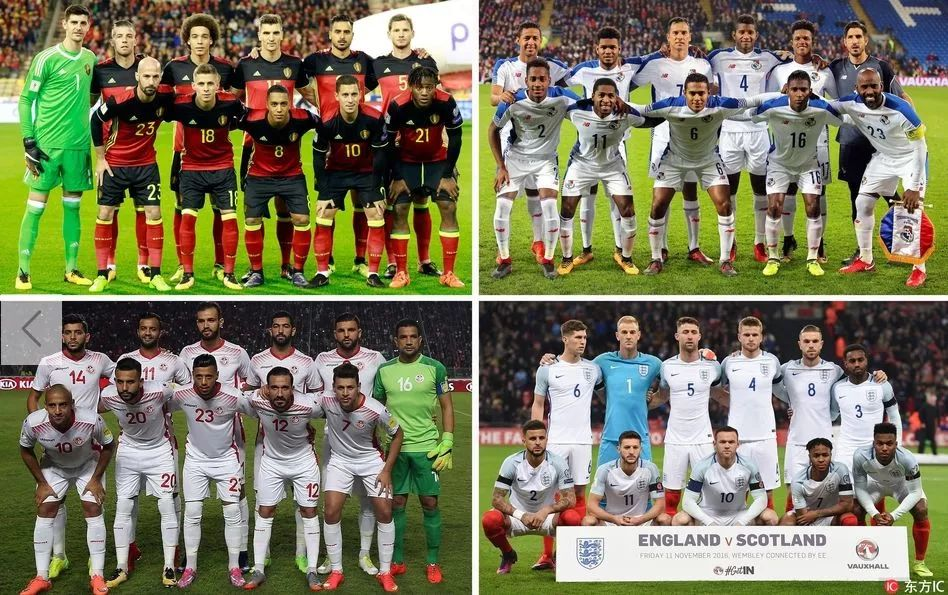后卫：阿诺德(利物浦)、卡希尔(切尔西)、菲尔-琼斯(曼联)、马奎尔(莱斯特城)、罗斯(热刺)、斯通斯(曼城)、特里皮尔(热刺)、沃克(曼城)、阿什利-扬(曼联)；

中场：阿里(热刺)、德尔夫(曼城)、戴尔(热刺)、亨德森(利物浦)、林加德(曼联)、洛夫图斯-奇克(水晶宫)；

前锋：凯恩(热刺)、拉什福德(曼联)、斯特林(曼城)、瓦尔迪(莱斯特城)、维尔贝克(阿森纳)。

门将：库尔图瓦(切尔西)、卡斯特尔斯(沃尔夫斯堡)、米尼奥莱(利物浦)、泽尔斯(安德莱赫特)

后卫：阿尔德韦雷尔德(热刺)、博亚塔(凯尔特人)、卡巴瑟利(沃特福德)、孔帕尼(曼城)、若尔丹-卢卡库(拉齐奥)、默尼耶(巴黎圣日耳曼)、维尔马伦(巴塞罗那)、维尔通亨(热刺)、西曼(洛杉矶FC)

中场：沙兹利(西布朗)、德布劳内(曼城)、登贝莱(热刺)、登东克尔(安德莱赫特)、费莱尼(曼联)、蒂勒芒斯(摩纳哥)、维特塞尔(天津权健)、贾努扎伊(皇家社会)

前锋：巴舒亚伊(切尔西/多特蒙德)、本特克(水晶宫)、卡拉斯科(大连一方)、阿扎尔(切尔西)、索尔根-阿扎尔(门兴)、卢卡库(曼联)、梅尔滕斯(那不勒斯)。

门将：何塞-卡尔德隆(乔里诺)、佩内多(布加勒斯特迪纳摩)、阿莱士-罗德里格斯(圣弗朗西斯科)；

后卫：阿里亚诺(爱国者)、巴洛伊(穆尼斯帕尔)、卡明斯(圣何塞地震)、埃里克-戴维斯(杜纳斯卡斯特雷达)、埃斯科瓦尔(纽约红牛)、马查多(休斯顿迪纳摩)、迈克尔-穆里略(纽约红牛)、奥瓦列(奥林匹亚)、弗朗西斯科-帕拉西奥斯(圣弗朗西斯科)、理查德-佩拉尔塔(亚利桑那)、罗曼-托雷斯(西雅图海湾人)；

中场：阿维拉(根特)、巴尔塞纳斯(阿尔塔米拉)、布伊特拉戈(穆尼斯帕尔)、卡马戈(圣马丁大学)、卡拉斯基利亚(陶罗)、库珀(智利大学)、戈多伊(圣何塞地震)、加布里埃尔-戈麦斯(布卡拉曼加)、何塞-冈萨雷斯(哥梅里卡奥)、克里斯蒂安-马丁内斯(哥伦布机员)、皮门特尔(阿玛多尔广场)、阿尔伯托-金特罗(利马大学)、何塞-罗德里格斯(根特)；

前锋：阿罗约(阿拉胡埃伦斯)、罗兰多-布莱克本(乔里诺)、伊斯梅尔-迪亚斯(拉科鲁尼亚)、法哈多(独立)、纳斯(扎卡特卡斯)、布拉斯-佩雷斯(穆尼斯帕尔)、特哈达(体育生)、加布里埃尔-托雷斯(瓦奇巴托)。

门将：马斯卢西(阿尔巴坦)、本-切里法(突尼斯希望)、本-穆斯塔法(阿尔沙巴布)、哈桑(沙托鲁)；

后卫：哈姆迪(扎马莱克)、布龙(根特)、贝多瓦(沙希尔星)、贝纳鲁亚内(莱斯特城)、本-优素福(卡斯帕萨)、莫里亚(斯法西恩)、莫斯尼(邓迪联)、切马姆(突尼斯希望)、哈达迪(第戎)、马劳尔(开罗国民)；

中场：斯希里(蒙彼利埃)、本-阿莫尔(吉达国民)、查拉利(突尼斯希望)、拉里比(切塞纳)、萨西(阿尔纳斯尔)、哈利勒(非洲俱乐部)、哈奥(特鲁瓦)、拉尔比(图尔)；

前锋：F-本-优素福(伊蒂法克)、巴德里(突尼斯希望)、斯哈菲(尼斯)、阿凯希(吉达联合)、哈兹里(雷恩)、斯利蒂(第戎)、哈利法(非洲俱乐部)。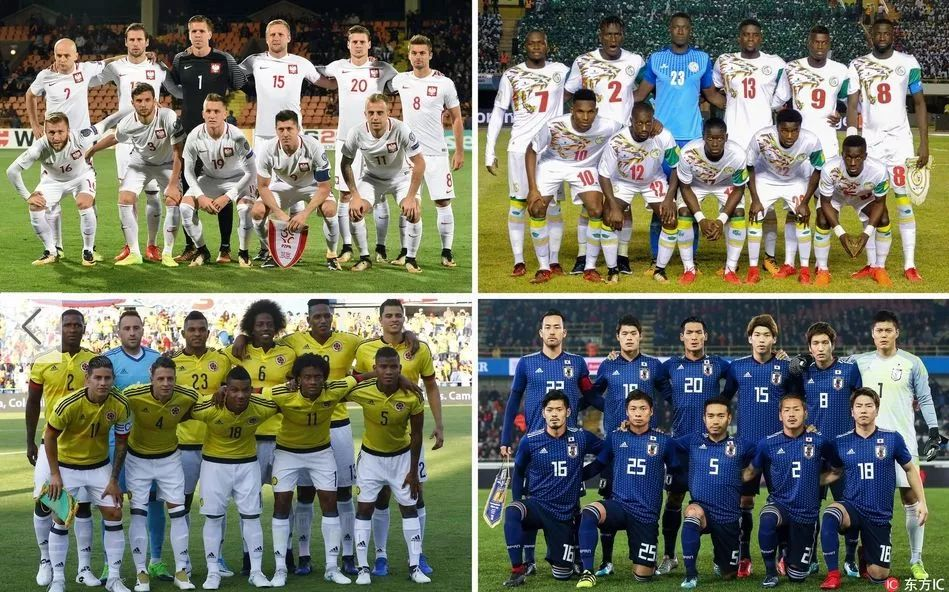门将：比亚科斯基(伊普斯维奇)、斯科鲁普斯基(罗马)、法比安斯基(斯旺西)、什琴斯尼(尤文图斯)；

后卫：贝德纳雷克(南安普顿)、卡明斯基(斯图加特)、贝雷辛斯基(桑普多利亚)、科济奥拉(基辅迪纳摩)、乔内克(斯帕尔)、帕兹丹(华沙莱吉亚)、格利克(摩纳哥)、皮什切克(多特蒙德)、耶德泽齐克(华沙莱吉亚)；

中场：利内蒂(桑普多利亚)、达维多维奇(巴勒莫)、弗兰科夫斯基(乔治罗尼亚)、马辛斯基(华沙莱吉亚)、格拉尔斯基(卢多戈雷茨)、佩斯科(格但斯克莱吉亚)、格罗西茨基(赫尔城)、里布斯(莫斯科火车头)、卡齐奥尔(戈尔尼克扎布热)、西曼斯基(华沙莱吉亚)、克里霍维亚克(西布朗)、泽林斯基(那不勒斯)、库尔扎瓦(戈尔尼克扎布热)、祖科夫斯基(戈尔尼克扎布热)；

前锋：科夫纳茨基(桑普多利亚)、莱万多夫斯基(拜仁慕尼黑)、特奥多切克(安德莱赫特)、米利克(那不勒斯)、维尔切克(布隆德比)。

门将：阿尔沃莱达(班菲尔德)、何塞-夸德拉多(卡尔达斯)、奥斯皮纳(阿森纳)、卡米罗-巴尔加斯(卡利体育)；

后卫：阿里亚斯(埃因霍温)、法里德-迪亚斯(奥林匹亚)、埃斯皮诺萨(赫罗纳)、法布拉(博卡青年)、梅迪纳(蒙特雷)、米纳(巴塞罗那)、莫西卡(赫罗纳)、奥斯卡-穆里略(帕丘卡)、桑切斯(热刺)、特西略(圣菲独立)、萨帕塔(AC米兰)；

中场：阿吉拉尔(卡利体育)、维尔玛-巴里奥斯(博卡青年)、卡多纳(博卡青年)、夸德拉多(尤文图斯)、奎利亚尔(弗拉门戈)、莱尔马(莱万特)、莫雷诺(上海绿地申花)、塞巴斯蒂安-佩雷斯(博卡青年)、哈梅斯-罗德里格斯(拜仁慕尼黑)、卡洛斯-桑切斯(西班牙人)、乌里韦(墨西哥美洲)；

前锋：巴卡(比利亚雷亚尔)、博尔哈(帕尔梅拉斯)、查拉(青年竞技)、法尔考(摩纳哥)、古铁雷斯(青年竞技)、伊斯基埃尔多(布莱顿)、穆里尔(塞维利亚)、金特罗(河床)、杜万-萨帕塔(桑普多利亚)。

门将：K-恩迪亚耶(迪亚拉夫达卡)、A-戈米(斯帕尔)、迪亚洛(雷恩)；

后卫：加萨马(阿拉尼亚体育)、瓦格(欧本)、萨里夫-西斯(瓦朗谢讷)、萨巴利(波尔多)、库利巴利(那不勒斯)、萨里夫-萨内(汉诺威96)、库亚特(西汉姆联)、姆博吉(扎尔吉里斯)；

中场：格耶(埃弗顿)、恩多耶(伯明翰)、阿尔弗雷德-恩迪亚耶(狼队)、巴杜-恩迪亚耶(斯托克城)；

前锋：索乌(布尔萨体育)、科纳特(亚眠)、迪亚夫拉-萨科(雷恩)、马内(利物浦)、伊斯梅拉-萨尔(雷恩)、迪乌夫(斯托克城)、尼昂(都灵)、凯塔-巴尔德(摩纳哥)。

后卫：长友佑都(加拉塔萨雷)、槙野智章(浦和红钻)、吉田麻也(南安普顿)、酒井宏树(马赛)、酒井高德(汉堡)、昌子源(鹿岛鹿角)、远藤航(浦和红钻)、植田直通(鹿岛鹿角)

中场：长谷部诚(法兰克福)、青山敏弘(广岛三箭)、本田圭佑(帕丘卡)、乾贵士(埃瓦尔)、香川真司(多特蒙德)、山口萤(大阪樱花)、宇佐美贵史(杜塞尔多夫)、原口元气(杜塞尔多夫)、柴崎岳(赫塔费)、大岛僚太(川崎前锋)、三竿健斗(鹿岛鹿角)、井手口阳介(莱昂文体)

前锋：冈崎慎司(莱斯特城)、大迫勇也(科隆/云达不莱梅)、武藤嘉纪(美因茨)、浅野拓磨(斯图加特)。